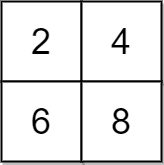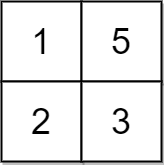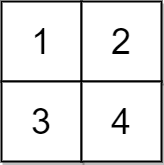2033. Minimum Operations to Make a Uni-Value Grid
Medium
479
38

You are given a 2D integer `grid` of size `m x n` and an integer `x`. In one operation, you can add `x` to or subtract `x` from any element in the `grid`.

A uni-value grid is a grid where all the elements of it are equal.

Return the minimum number of operations to make the grid uni-value. If it is not possible, return `-1`.

Example 1:```Input: grid = [[2,4],[6,8]], x = 2
Output: 4
Explanation: We can make every element equal to 4 by doing the following:
- Add x to 2 once.
- Subtract x from 6 once.
- Subtract x from 8 twice.
A total of 4 operations were used.
```

Example 2:```Input: grid = [[1,5],[2,3]], x = 1
Output: 5
Explanation: We can make every element equal to 3.
```

Example 3:```Input: grid = [[1,2],[3,4]], x = 2
Output: -1
Explanation: It is impossible to make every element equal.
```

Constraints:

• `m == grid.length`
• `n == grid[i].length`
• `1 <= m, n <= 105`
• `1 <= m * n <= 105`
• `1 <= x, grid[i][j] <= 104`
Accepted
19.3K
Submissions
36.8K
Acceptance Rate
52.3%

Seen this question in a real interview before?
1/4
Yes
No

Discussion (0)

Related Topics